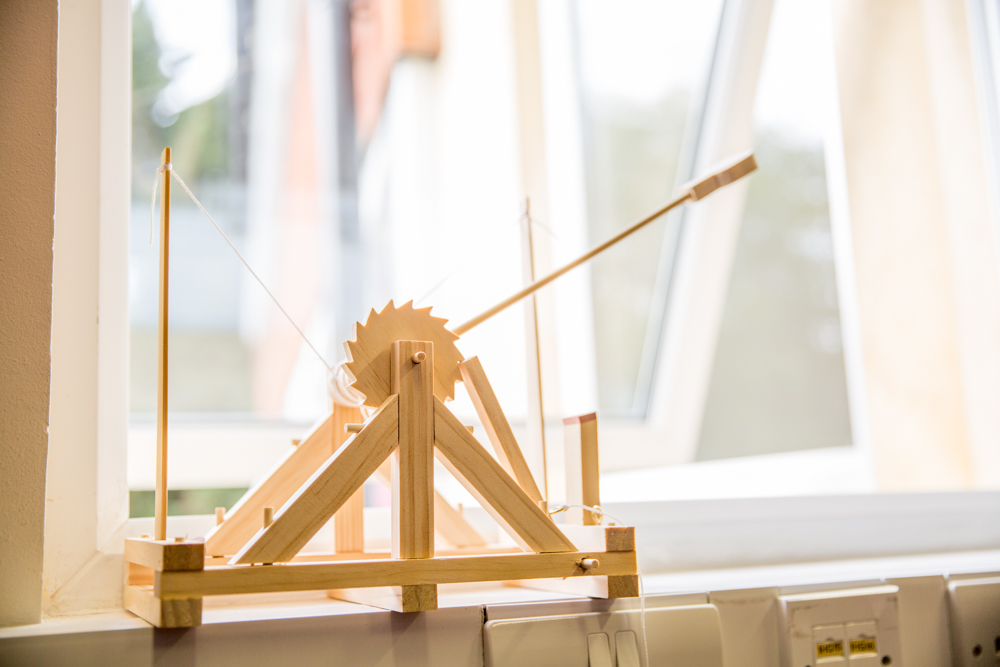• ""

# MathematicsMathematics combines well with many other A level subjects, especially the sciences and Economics. The key requirements are enthusiasm for problem solving, perseverance, good ability in algebra and at least grade 7 pass in the subject at Higher Tier GCSE (grade 9 for Further Mathematics or, exceptionally, grade 8 may be acceptable).

There is also an opportunity for studying Further Mathematics, which provides an excellent foundation for the study of Mathematics at university, or for Engineering, Science and Computer Science students with aspirations to Oxford and Cambridge.

While studying Mathematics you will be expected to:

•  use mathematical skills and knowledge to solve problems
• solve quite complicated problems by using mathematical arguments and logic
• simplify real life situations so that you can use mathematics to show what is happening and what might happen in different circumstances
• use the Mathematics that you learn to solve problems that are given to you in a real life context use calculator technology and other resources (such as formulae booklets or statistical tables) effectively and appropriately; understand when not to use such technology, and its limitations

Mathematics at A level is demanding, but also very rewarding. A level Mathematics is a much sought-after qualification for entry to a wide variety of university courses.

Pure Mathematics
Often referred to as Core Maths, this extends your knowledge of such topics as algebra and trigonometry, and introduces new concepts such
as calculus.

Mechanics
You will learn how to describe mathematically the motion of objects and how they respond to forces acting upon them. You will learn the technique of mathematical modelling; that is, of turning a complicated physical problem into a simpler one that can be analysed and solved using mathematical methods.

Statistics
You will learn how to analyse and summarise numerical data in order to arrive at conclusions about it. Many of the concepts have applications in a wide area of other fields – from assessing what your car insurance is likely to cost to how likely it is for the Earth to be hit by a comet in the next few years.

Decision Mathematics
You will learn how to solve problems involving networks. Many of these problems and their algorithms are of recent origin and give an insight into the huge amount of Mathematics used in computing.